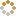﻿ Ningbo University of Technology Online Judge ::  A+B Problem
• ###  A+B Problem

• 时间限制: 1000 ms　内存限制: 1024 K
• 问题描述
• Calculate a + b

note: a and b may bigger than INT_MAX

• 输入
• The input will consist of a series of pairs of integers a and b,separated by a space, one pair of integers per line, 0 0 means the end of the input, and do not need to output.
• 输出
• For each pair of input integers a and b you should output the sum of a and b in one line,and with one line of output for each line in input.
• 样例输入
• ```1 5
0 0
```
• 样例输出
• ```6
```
• 提示
• `无`
• 来源
• `本站或者转载`
• 操作
﻿